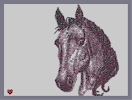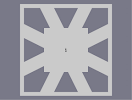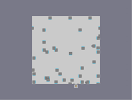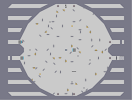### My Little PonyHover over the thumbnail for a full-size version.

Author wedgie123 author:wedgie123 bitesized n-art rated 2006-07-19 4 by 158 people. \$My Little Pony#wedgie123#none#00000000000000000000000000000000000000000000000000000000000000000000000000000000000000000000000000000000000000000000000000000000000000000000000000000000000000000000000000000000000000000000000000000000000000000000000000000000000000000000000000000000000000000000000000000000000000000000000000000000000000000000000000000000000000000000000000000000000000000000000000000000000000000000000000000000000000000000000000000000000000000000000000000000000000000000000000000000000000000000000000000000000000000000000000000000000000000000000000000000000000000000000000000000000000000000000000000000000000000000000000000000000000000000000000000000000000000000000000000000000000000000000000000000000000000000000000000000000000000|5^490,120!5^474,68!5^474,60!5^474,60!10^348,48!10^470,31!10^331,262!10^364,378!3^457,476!3^479,431!3^476,573!3^482,517!3^498,567!10^500,112!10^455,92!10^459,117!10^427,110!10^400,136!10^378,101!10^340,117!10^353,150!3^379,193!10^515,114!10^534,125!10^562,136!10^595,165!10^649,244!10^687,318!10^707,395!10^676,455!10^644,486!10^586,515!10^491,423!10^523,400!10^534,373!10^536,338!10^527,302!10^517,266!10^471,41!10^467,49!10^465,58!10^461,69!10^457,78!10^456,86!10^456,98!10^457,107!10^458,112!10^474,37!10^477,44!10^479,49!10^484,57!10^488,66!10^490,70!10^492,76!10^494,80!10^495,88!10^496,94!10^496,101!10^496,107!10^496,114!10^496,123!10^496,129!10^495,137!10^495,143!10^496,148!10^489,156!10^483,152!10^476,145!10^467,136!10^458,128!10^473,53!10^477,58!10^481,67!10^484,73!10^484,78!10^486,86!10^487,92!10^487,98!10^487,105!10^487,113!10^487,120!10^488,127!10^488,132!10^488,139!10^488,144!10^492,81!10^448,115!10^442,115!10^437,113!10^430,111!10^419,109!10^413,115!10^413,120!10^415,126!10^399,137!10^394,133!10^388,124!10^386,118!10^382,112!10^381,108!10^374,95!10^369,86!10^365,81!10^359,70!10^354,64!10^349,58!10^348,55!10^343,61!10^339,68!10^337,77!10^336,86!10^333,94!10^334,102!10^337,110!10^343,125!10^347,134!10^348,141!10^351,147!10^358,160!10^356,157!10^374,172!10^368,168!10^362,165!9^334,172,0,1,0,10,0,-1,0!9^328,200,0,1,0,12,0,-1,0!9^346,162,0,1,0,10,0,-1,0!9^349,183,0,1,0,11,0,-1,0!9^337,169,0,1,0,11,0,-1,0!9^341,165,0,1,0,9,0,-1,0!9^351,160,0,1,0,7,0,-1,0!9^331,196,0,1,0,9,0,-1,0!9^335,192,0,1,0,8,0,-1,0!9^338,189,0,1,0,9,0,-1,0!9^340,186,0,1,0,8,0,-1,0!9^343,183,0,1,0,8,0,-1,0!9^350,180,0,1,0,9,0,-1,0!9^355,175,0,1,0,8,0,-1,0!9^359,173,0,1,0,8,0,-1,0!9^355,164,0,1,0,7,0,-1,0!9^348,164,0,1,0,8,0,-1,0!9^344,166,0,1,0,7,0,-1,0!9^354,182,0,1,0,9,0,-1,0!9^359,179,0,1,0,8,0,-1,0!9^364,176,0,1,0,8,0,-1,0!9^350,176,0,1,0,9,0,-1,0!9^368,174,0,1,0,8,0,-1,0!9^346,179,0,1,0,7,0,-1,0!3^377,184!3^375,174!3^372,196!3^367,203!3^360,212!3^357,223!3^350,230!3^344,237!10^343,237!10^338,241!10^334,248!10^332,252!3^352,281!3^354,290!3^357,300!3^358,314!3^358,329!3^358,345!3^361,357!3^365,364!3^366,373!3^365,381!3^362,399!3^358,418!3^356,435!3^350,450!3^343,464!3^441,515!3^446,502!3^450,491!3^455,478!3^456,451!3^462,439!3^472,430!3^360,409!3^364,389!3^356,427!3^353,443!3^347,457!3^358,335!3^358,319!3^383,294!3^366,437!3^384,308!3^383,323!3^386,343!3^384,361!3^381,384!3^375,407!3^371,422!9^334,261,0,1,0,15,0,-1,0!9^347,281,0,1,0,12,0,-1,0!9^345,268,0,1,0,13,0,-1,0!9^349,271,0,1,0,13,0,-1,0!9^342,266,0,1,0,11,0,-1,0!9^338,264,0,1,0,11,0,-1,0!9^352,275,0,1,0,11,0,-1,0!9^352,278,0,1,0,13,0,-1,0!9^345,279,0,1,0,11,0,-1,0!9^343,275,0,1,0,10,0,-1,0!9^341,271,0,1,0,9,0,-1,0!9^335,266,0,1,0,10,0,-1,0!10^368,284!10^371,297!10^371,308!10^372,315!10^372,325!10^369,333!10^369,344!10^368,352!10^366,362!10^366,243!10^364,258!10^365,274!10^369,349!10^369,341!10^369,328!10^370,319!10^370,312!10^370,301!10^368,290!10^366,279!10^366,267!10^370,305!10^368,290!10^367,335!10^355,428!10^357,420!10^361,408!10^367,397!10^370,380!10^366,370!10^367,368!10^367,358!10^367,352!10^370,333!10^362,352!10^362,338!10^362,329!10^362,325!10^367,331!10^366,318!10^366,308!10^354,267!10^350,261!10^347,259!10^339,254!10^334,252!10^355,259!10^355,257!10^352,249!10^350,241!10^349,241!10^349,252!10^345,252!10^344,252!10^336,247!10^344,241!10^348,241!10^343,246!10^350,235!10^358,227!10^459,251!10^462,241!10^460,236!10^455,226!10^449,218!10^438,212!10^450,238!10^444,232!10^434,231!10^427,231!10^423,234!10^415,270!10^419,247!10^422,237!10^410,243!10^421,213!10^421,197!10^405,222!10^413,233!10^419,222!10^431,221!10^437,221!10^448,226!10^457,231!10^461,250!10^460,245!10^454,240!10^451,228!10^450,226!10^459,238!10^459,244!10^462,232!10^458,226!10^452,215!10^443,203!10^433,199!10^405,200!10^428,208!10^413,212!10^417,244!10^417,235!10^417,231!10^419,223!10^427,214!10^421,215!10^414,233!10^413,243!10^413,247!10^413,250!10^415,226!10^411,216!10^431,207!10^412,153!10^407,155!10^402,158!10^395,165!10^389,167!10^381,171!10^375,173!10^368,174!10^382,169!10^398,160!10^407,155!5^445,133!5^430,134!5^423,136!5^413,140!5^405,147!5^399,151!5^392,153!5^376,156!5^368,153!5^359,147!5^353,141!5^347,125!5^345,119!5^342,108!5^341,93!5^341,83!5^344,73!5^347,68!5^356,73!5^369,90!5^381,109!5^384,118!5^390,135!5^393,146!5^393,148!5^391,149!5^379,136!5^377,130!5^373,120!5^370,111!5^365,95!5^362,80!5^353,72!5^353,72!5^379,99!5^384,111!5^381,124!5^376,115!5^371,107!5^367,100!5^359,91!5^353,84!5^352,83!5^351,95!5^351,106!5^356,118!5^370,136!5^373,147!5^363,135!5^359,131!5^351,122!5^347,112!5^343,99!5^343,94!5^343,85!5^351,90!5^357,112!5^365,127!5^360,120!5^356,113!5^355,103!5^363,112!5^374,138!5^365,114!5^359,102!5^359,98!5^373,117!5^384,136!5^390,150!5^386,153!5^382,153!5^374,147!5^362,135!5^353,127!5^360,145!5^364,147!5^374,160!5^393,151!5^413,144!5^415,141!5^417,140!5^430,136!5^436,133!5^438,132!5^441,121!5^439,119!5^428,117!5^421,115!5^417,118!5^417,126!5^419,129!5^422,119!5^436,120!5^445,123!5^458,124!5^441,127!5^428,127!5^420,132!5^478,126!5^476,125!5^469,121!5^468,119!5^466,114!5^466,108!5^467,106!5^471,117!5^475,121!5^479,111!5^479,107!5^478,92!5^477,86!5^476,75!5^474,69!5^470,75!5^468,82!5^464,96!5^469,102!5^470,97!5^469,92!5^467,88!5^467,79!5^477,71!5^481,102!5^482,116!10^366,171!10^360,175!10^351,177!10^352,161!10^373,160!10^373,158!10^403,149!10^416,148!10^432,145!10^458,142!10^455,134!10^473,128!10^487,132!10^486,134!10^468,139!10^457,130!10^451,120!10^451,120!9^401,129,0,1,0,9,0,-1,0!9^401,125,0,1,0,6,0,-1,0!9^399,121,1,1,16,0,0,0,-1!9^399,117,1,1,16,0,0,0,-1!9^400,114,1,1,16,0,0,0,-1!9^402,109,1,1,17,0,0,0,-1!9^406,105,1,1,17,0,0,0,-1!9^411,105,1,1,18,0,0,0,-1!9^419,109,1,1,18,0,0,0,-1!9^416,108,1,1,19,0,0,0,-1!10^509,229!10^512,215!10^510,207!10^506,199!10^500,190!10^548,329!10^550,315!10^550,310!10^550,305!10^549,295!10^546,282!10^538,260!10^537,254!10^544,326!10^544,317!10^544,313!10^544,305!10^542,297!10^537,291!10^541,291!10^538,278!10^535,273!10^531,252!10^541,288!10^542,303!10^535,285!10^534,275!10^534,263!10^533,257!10^542,275!10^545,287!10^543,283!10^539,267!10^533,251!10^530,243!10^531,240!10^531,234!10^532,227!3^519,230!3^521,219!3^520,213!3^512,193!3^505,181!3^503,171!3^501,151!3^516,202!3^500,226!3^492,226!3^485,227!3^472,235!3^467,226!3^462,221!3^457,212!3^451,205!3^434,196!3^462,222!3^451,211!3^463,203!3^474,215!3^472,219!3^464,206!3^480,223!3^464,211!3^446,195!3^504,216!3^495,208!3^485,202!3^473,188!3^467,177!3^490,213!3^478,205!3^505,201!3^461,258!3^463,251!3^464,243!3^467,235!3^472,229!3^472,229!3^460,255!3^472,156!3^491,175!3^501,185!3^484,163!3^467,153!3^453,149!3^446,146!3^455,195!3^444,187!3^438,185!3^424,185!3^403,198!3^423,176!3^443,177!3^456,183!3^463,195!3^416,204!3^413,190!3^435,179!3^435,164!3^454,163!10^407,177!10^410,172!10^412,167!10^413,165!10^416,162!10^420,156!10^422,152!10^426,145!10^427,144!10^416,157!10^412,164!10^489,119!10^489,110!10^489,103!10^487,91!10^471,64!10^469,57!10^466,59!10^462,66!10^461,74!10^461,88!10^461,100!10^461,110!10^466,120!10^466,123!10^348,66!10^359,76!10^366,85!10^370,92!10^375,99!10^380,109!10^382,117!10^369,127!10^360,115!10^351,102!10^347,97!10^345,84!10^346,81!10^375,147!10^372,148!10^414,130!3^358,232!3^363,244!3^364,252!3^373,233!3^383,228!3^390,220!3^402,200!3^373,220!3^396,209!3^399,260!3^382,260!3^375,273!3^376,283!3^376,257!3^392,246!3^403,241!3^398,229!3^379,244!3^389,414!3^395,399!3^399,387!3^402,366!3^407,344!3^410,316!3^410,303!3^413,288!3^413,274!3^410,265!3^407,253!3^414,260!3^423,254!3^430,250!3^411,324!3^404,354!3^401,382!3^402,383!3^387,404!3^395,392!3^399,372!3^405,353!3^410,331!3^398,368!3^402,334!3^407,318!3^404,302!3^406,292!3^406,277!3^403,308!3^405,324!3^410,285!3^404,343!3^397,352!3^397,319!3^397,287!3^398,267!3^404,335!3^406,358!3^398,379!3^389,405!3^398,398!3^393,408!3^385,420!3^406,312!3^408,299!3^408,290!3^408,273!3^409,253!3^402,277!3^400,300!3^398,342!3^393,364!3^390,383!3^384,398!3^400,327!3^374,335!3^373,343!3^373,357!3^373,368!3^370,385!3^369,368!3^370,352!3^375,324!3^376,305!3^376,291!3^372,268!3^367,260!3^380,317!3^379,334!3^374,356!3^378,262!3^371,242!3^395,338!3^387,374!3^376,401!3^390,353!3^389,389!3^370,413!3^365,425!3^360,476!3^355,470!3^358,460!3^358,448!3^348,468!3^343,472!3^340,480!3^337,483!3^339,473!3^353,479!3^362,485!3^350,484!3^422,288!3^419,300!3^418,325!3^420,314!3^418,304!3^421,272!3^412,336!3^412,352!3^409,376!3^369,185!3^367,194!3^360,203!3^353,214!3^349,225!3^362,183!3^354,183!3^372,181!10^489,146!10^479,139!10^476,137!10^463,129!10^449,129!3^452,261!3^451,252!3^446,244!3^438,237!3^428,244!3^438,245!3^430,237!3^422,263!3^435,261!3^446,267!3^418,286!3^433,285!3^426,274!3^438,235!3^432,224!3^421,224!3^446,420!3^446,420!3^444,405!3^444,397!3^444,385!3^447,375!3^447,371!3^448,366!3^443,415!3^417,383!3^418,364!3^402,415!3^406,402!3^413,391!3^403,387!3^398,411!3^420,344!3^420,333!10^484,361!10^493,357!10^498,355!10^505,351!10^508,344!10^512,338!10^512,333!10^512,327!10^512,322!10^512,318!10^511,301!10^511,293!10^506,336!10^497,407!10^504,402!10^506,401!10^511,397!10^514,395!10^521,389!10^521,386!10^523,384!10^526,379!10^529,374!10^532,371!10^532,366!10^532,363!10^535,370!10^539,359!10^543,353!10^545,335!10^545,335!10^539,344!10^537,352!10^529,364!10^521,378!10^512,388!10^498,398!10^494,401!10^502,392!10^516,376!10^523,369!10^527,363!10^535,348!10^535,342!10^535,354!10^533,382!10^531,386!10^528,393!10^524,397!10^520,399!10^511,405!10^508,406!10^504,408!10^497,411!10^492,413!10^486,418!10^483,419!10^486,411!10^486,406!10^497,424!10^501,418!10^507,413!10^514,408!10^519,403!10^526,392!10^532,377!10^535,361!10^512,394!10^502,403!10^491,413!10^482,422!10^476,424!10^530,314!10^532,334!10^530,320!10^529,319!10^522,303!10^520,292!10^519,283!10^531,328!10^527,309!10^509,308!10^446,411!10^446,419!10^446,435!10^445,426!10^402,544!10^401,547!10^390,547!10^383,545!10^335,485!10^350,517!10^350,513!10^349,508!10^348,506!10^345,503!10^344,500!10^341,497!10^339,495!10^339,495!10^430,513!10^426,515!10^418,515!10^416,514!10^410,511!10^406,507!10^401,456!10^400,466!10^400,471!10^398,477!10^396,481!10^394,487!10^394,491!10^428,524!10^426,527!10^423,530!10^418,535!10^413,540!10^409,541!10^404,541!3^436,526!3^415,547!3^419,545!3^423,543!3^426,542!3^430,538!3^433,531!3^407,548!10^431,521!10^428,525!10^426,528!10^421,533!10^417,535!10^411,535!10^402,541!10^392,545!10^409,529!10^394,532!10^381,536!10^394,537!10^388,538!10^385,538!10^379,536!10^370,533!10^363,529!10^360,527!10^356,524!10^417,526!10^413,526!10^402,527!10^394,527!10^383,527!10^372,527!10^369,527!10^364,523!10^368,520!10^391,534!10^383,524!10^376,524!10^386,527!10^408,524!10^412,519!10^409,517!10^399,515!10^394,515!10^386,515!10^376,515!10^367,515!10^363,515!10^364,523!10^377,521!10^388,517!10^400,517!10^345,509!10^341,504!10^367,507!10^378,507!10^389,507!10^394,507!10^403,508!10^363,510!10^363,504!10^360,500!10^357,494!10^355,488!10^350,485!10^353,492!10^348,487!10^400,533!10^400,532!10^408,531!10^399,522!10^392,520!10^387,534!10^376,534!10^369,535!10^367,524!10^375,522!10^375,517!10^378,512!10^433,490!10^433,484!10^430,479!10^428,477!10^425,474!10^421,474!10^425,458!10^419,457!10^416,457!10^412,457!10^409,457!10^406,453!10^435,465!10^430,462!10^426,460!10^422,460!10^415,459!10^402,467!10^403,473!10^403,478!10^400,484!10^399,486!10^399,490!10^399,492!10^399,498!10^399,499!10^403,503!10^405,507!10^424,500!10^423,502!10^420,500!10^416,498!10^413,494!10^413,489!10^413,484!10^422,492!10^419,488!10^418,486!10^417,472!10^417,476!10^433,497!3^440,511!3^450,485!3^435,507!3^442,485!3^442,477!3^441,471!3^440,471!3^441,491!3^443,477!3^446,467!3^452,461!3^458,464!3^457,436!3^465,429!3^473,424!3^389,505!3^379,499!3^372,499!3^359,496!3^367,498!3^387,502!3^390,494!3^382,492!3^376,491!3^369,488!3^371,482!3^385,482!3^394,473!3^372,469!3^389,464!3^375,456!3^388,448!3^394,430!3^430,453!3^421,448!3^415,442!3^412,440!3^408,434!3^407,432!3^402,425!3^402,434!3^408,446!3^420,453!3^429,455!3^413,445!3^406,440!3^400,436!3^400,442!3^400,447!3^398,455!3^392,466!3^391,479!3^448,440!3^456,419!3^454,402!3^455,382!3^455,373!3^451,396!3^451,415!3^451,438!3^453,391!3^443,389!3^442,400!3^463,397!3^463,381!3^464,369!3^464,415!3^475,380!3^472,409!3^460,352!3^449,352!3^453,340!3^454,359!3^453,362!3^438,448!3^449,459!3^440,455!3^438,452!3^438,442!3^438,431!3^436,420!3^435,411!3^435,398!3^447,445!3^451,456!3^378,433!3^426,436!3^413,420!3^421,435!3^427,443!3^425,416!3^423,405!3^430,371!3^520,165!3^520,180!3^520,193!3^523,205!3^527,216!3^510,172!3^507,161!9^502,249,1,1,16,0,0,0,-1!9^481,263,1,1,18,0,0,0,-1!9^499,265,1,1,19,0,0,0,-1!9^503,262,1,1,20,0,0,0,-1!9^504,258,1,1,17,0,0,0,-1!9^505,253,1,1,20,0,0,0,-1!9^495,266,1,1,21,0,0,0,-1!9^490,266,1,1,21,0,0,0,-1!9^484,265,1,1,22,0,0,0,-1!9^481,260,1,1,23,0,0,0,-1!9^499,248,1,1,25,0,0,0,-1!9^495,251,1,1,22,0,0,0,-1!9^499,254,1,1,22,0,0,0,-1!9^497,257,1,1,22,0,0,0,-1!9^499,261,1,1,23,0,0,0,-1!9^495,263,1,1,23,0,0,0,-1!9^486,261,1,1,22,0,0,0,-1!9^490,260,1,1,22,0,0,0,-1!9^486,258,1,1,23,0,0,0,-1!9^492,258,1,1,24,NaN,0,0,NaN!9^488,248,1,1,20,0,0,0,-1!9^493,246,1,1,20,0,0,0,-1!9^497,246,1,1,22,0,0,0,-1!9^483,254,1,1,21,0,0,0,-1!9^484,249,1,1,21,0,0,0,-1!9^488,272,1,1,20,0,0,0,-1!9^484,272,1,1,22,0,0,0,-1!9^478,270,1,1,21,0,0,0,-1!9^475,268,1,1,21,0,0,0,-1!9^504,240,1,1,21,0,0,0,-1!9^507,244,1,1,21,0,0,0,-1!9^499,239,1,1,21,0,0,0,-1!9^496,239,1,1,22,0,0,0,-1!9^492,239,1,1,23,0,0,0,-1!10^507,240!10^506,232!10^506,237!10^506,223!10^508,214!10^509,202!10^509,193!10^503,181!10^507,216!10^504,226!10^506,207!10^506,187!10^502,180!10^503,233!10^494,231!10^488,233!10^483,235!10^472,243!10^490,231!10^498,229!10^474,239!10^476,273!10^482,272!3^516,272!3^516,277!3^517,292!3^522,302!3^530,319!3^530,344!3^531,353!3^482,351!3^492,346!3^501,341!3^501,328!3^502,308!3^497,307!3^492,315!3^486,322!3^482,329!3^476,342!3^475,345!3^475,352!3^475,363!3^477,356!3^477,333!3^486,338!3^492,334!3^498,318!3^500,300!3^505,287!3^509,280!3^513,271!3^509,294!3^509,319!3^497,283!3^491,287!3^480,294!3^470,306!3^462,321!3^460,339!3^456,358!3^453,291!3^445,303!3^437,318!3^426,330!3^418,339!3^434,308!3^437,291!3^428,317!3^445,288!3^453,275!3^460,293!3^438,354!3^429,366!3^463,334!3^468,294!10^504,113!10^509,113!10^522,117!10^525,121!10^530,126!10^690,313!10^692,327!10^695,334!10^696,342!10^696,351!10^700,360!10^701,370!10^705,378!10^706,387!10^706,389!10^702,377!10^533,125!10^539,127!10^545,129!10^553,135!10^555,136!10^561,140!10^565,145!10^569,153!10^570,161!10^568,151!10^575,154!10^579,159!10^584,163!10^587,164!10^591,167!10^592,168!10^595,172!10^596,177!10^597,184!10^597,190!10^605,178!10^608,180!10^612,182!10^615,187!10^619,193!10^619,198!10^619,209!10^619,219!10^619,227!10^619,237!10^616,248!10^614,258!10^622,201!10^627,208!10^629,210!10^630,211!10^632,212!10^635,217!10^639,221!10^645,225!10^647,231!10^648,237!10^649,244!10^649,252!10^649,264!10^639,275!10^626,293!10^618,315!10^653,242!10^653,245!10^653,253!10^653,257!10^658,254!10^658,255!10^661,261!10^661,272!10^660,279!10^653,294!10^648,307!10^643,322!10^654,285!10^661,286!10^661,286!10^672,293!10^675,297!10^680,303!10^686,310!10^689,323!10^664,291!10^708,400!10^708,406!10^708,413!10^707,416!10^705,420!10^702,427!10^699,435!10^697,439!10^694,442!10^690,446!10^687,449!10^684,453!10^681,455!10^678,455!10^673,457!10^669,460!10^663,466!10^658,471!10^652,476!10^648,480!10^643,485!10^638,491!10^636,489!10^629,496!10^622,507!10^619,518!10^619,525!10^619,534!10^621,540!10^624,543!10^628,547!10^635,549!10^636,549!10^641,548!10^644,546!10^649,543!10^649,542!10^652,538!10^652,536!10^652,531!10^638,477!10^628,483!10^625,483!10^620,487!10^614,492!10^609,495!10^603,500!10^597,503!10^591,505!10^583,508!10^580,510!10^577,515!10^567,526!10^563,532!10^560,536!10^559,540!10^559,543!10^559,551!10^559,553!10^573,519!10^562,546!10^565,548!10^572,550!10^580,550!10^586,550!10^558,542!10^557,558!10^558,566!10^566,569!10^570,572!10^578,577!10^589,578!10^598,577!10^606,575!10^608,573!10^610,571!10^612,569!10^489,430!10^491,474!10^489,437!10^490,443!10^491,450!10^491,458!10^491,465!10^490,473!10^490,461!10^490,454!10^490,442!10^488,431!10^491,469!10^490,480!10^489,488!3^477,566!3^475,558!3^475,554!3^475,546!3^476,540!3^478,532!3^479,525!3^481,522!3^487,506!3^488,497!3^489,566!3^507,552!3^516,544!3^529,537!3^548,534!3^557,532!3^559,532!3^540,534!3^505,558!3^507,425!3^515,444!3^542,484!3^543,429!3^551,429!3^553,422!3^529,398!3^538,413!3^540,426!3^553,357!3^553,344!3^551,345!3^509,120!3^509,124!3^509,132!3^510,138!3^515,150!3^519,161!3^512,159!3^505,149!3^501,133!3^517,128!3^527,132!3^536,132!3^522,139!3^518,144!3^517,155!3^521,190!3^458,445!3^419,474!3^415,480!3^415,489!3^418,496!3^424,500!3^428,501!3^428,488!3^433,465!3^428,459!3^416,459!3^409,459!3^402,462!3^399,487!3^411,506!3^417,511!3^363,488!3^366,489!3^375,491!3^383,491!3^388,491!3^390,491!3^392,496!3^392,504!3^382,509!3^374,504!3^370,503!3^367,501!3^366,500!3^365,500!3^383,501!3^381,495!3^372,489!3^379,478!3^361,476!3^359,481!10^393,540!10^395,525!10^383,524!10^380,516!10^379,512!10^372,504!10^372,505!10^371,521!10^371,526!10^379,531!10^387,531!10^394,523!10^394,521!10^385,520!10^384,511!10^381,504!10^422,523!10^419,522!10^412,518!10^405,513!10^399,509!10^393,508!10^390,506!10^408,526!10^404,521!10^399,516!10^395,513!10^405,535!10^390,534!10^383,533!10^369,536!10^372,537!10^372,512!10^378,520!10^383,524!10^389,527!10^400,529!10^392,498!10^431,504!10^425,501!10^420,497!10^417,494!10^432,499!10^436,493!10^434,485!10^502,253!10^502,256!10^499,259!10^498,263!10^494,265!10^491,265!10^487,265!10^486,265!10^484,262!10^484,257!10^484,253!10^500,249!10^493,249!10^487,263!10^481,257!10^482,263!10^499,247!10^497,258!10^491,260!10^481,262!3^541,330!3^540,317!3^536,309!3^532,296!3^530,287!3^526,267!3^525,257!3^523,245!3^523,233!3^523,225!3^528,278!3^494,273!3^486,274!3^475,279!3^484,281!3^476,282!3^470,294!3^465,311!3^461,326!3^451,278!3^446,296!3^444,304!3^439,313!3^445,277!10^609,283!10^579,220!10^535,167!10^558,198!10^600,254!10^627,325!10^635,374!10^619,338!10^619,384!10^596,416!10^619,403!10^598,436!10^569,484!10^580,457!10^594,444!10^529,151!10^529,164!10^539,176!10^552,192!10^557,198!10^553,193!10^545,182!10^543,181!10^534,163!10^533,153!10^532,143!10^531,135!10^536,130!10^540,139!10^540,152!10^554,180!10^569,192!10^576,200!10^569,205!10^578,213!10^589,225!10^595,237!10^609,266!10^611,275!10^617,299!10^626,330!10^628,344!10^625,361!10^624,374!10^623,385!10^616,408!10^601,439!10^580,464!10^578,468!10^562,491!10^350,282!10^349,279!10^349,278!10^347,275!10^344,273!10^342,269!10^339,268!10^335,264!10^335,264!10^333,262!3^429,299!3^407,319!3^409,301!3^409,299!3^412,284!3^412,263!3^412,245!3^398,173!3^386,173!3^380,182!3^391,180!3^380,192!3^374,207!3^383,208!3^361,222!3^392,189!3^409,172!3^440,151!3^435,155!3^429,157!3^423,163!3^413,174!3^407,180!3^465,160!3^451,154!3^442,159!3^436,164!3^461,423!3^458,423!3^486,399!3^493,391!3^502,383!3^506,379!3^516,364!3^518,356!3^520,340!3^520,316!3^509,370!3^479,410!3^465,407!3^497,365!3^488,369!3^476,375!3^468,391!3^472,393!3^427,393!3^424,429!3^355,470!3^355,465!3^356,454!3^356,450!3^356,440!3^356,430!3^365,473!3^364,452!3^364,428!3^367,410!3^366,463!3^414,427!3^409,418!3^407,398!3^409,408!3^528,173!3^521,173!3^463,279!3^460,272!3^461,262!3^462,254!3^469,247!3^477,240!3^480,236!3^477,241!3^466,258!3^466,262!3^466,267!3^553,176!3^547,172!3^545,168!3^545,164!3^544,159!3^544,152!3^544,148!3^544,140!3^544,136!3^533,142!3^534,149!3^535,158!10^528,148!10^527,140!10^522,132!10^512,112!10^507,108!10^504,108!10^546,145!10^550,167!10^556,177!10^562,186!10^563,189!10^566,192!10^571,198!10^553,146!10^553,147!10^553,153!10^554,162!10^555,166!10^560,173!10^563,179!10^566,183!10^572,190!10^575,196!10^579,201!10^558,141!10^558,141!10^560,146!10^562,153!10^565,165!10^568,168!10^570,172!10^573,176!10^578,181!10^581,189!10^581,195!10^581,202!10^581,212!10^585,219!10^592,224!10^597,229!10^599,235!10^604,245!10^608,255!10^573,161!10^573,162!10^576,169!10^579,174!10^585,191!10^588,198!10^588,207!10^588,217!10^588,225!10^579,163!10^580,163!10^585,170!10^586,180!10^589,184!10^590,192!10^590,201!10^590,220!10^595,196!10^594,202!10^594,209!10^595,218!10^595,223!10^608,191!10^608,194!10^607,202!10^606,209!10^606,212!10^605,221!10^605,229!10^605,233!10^610,204!10^610,210!10^610,214!10^610,223!10^610,229!10^610,234!10^609,241!10^560,149!10^561,166!10^564,169!10^570,176!10^573,187!10^570,163!10^572,167!10^583,181!10^602,188!10^602,196!10^602,202!10^600,197!10^600,199!10^600,210!10^600,215!10^623,213!10^623,216!10^624,224!10^624,230!10^624,239!10^624,247!10^622,256!10^621,264!10^620,269!10^618,273!10^617,278!10^616,284!10^616,285!10^633,221!10^633,223!10^633,233!10^633,238!10^633,246!10^631,252!10^629,259!10^628,263!10^626,266!10^624,274!10^623,284!10^622,291!10^643,234!10^643,236!10^642,247!10^639,254!10^638,258!10^634,264!10^632,269!10^629,274!10^623,280!10^619,283!10^616,288!10^617,205!10^617,205!10^616,219!10^616,226!10^613,234!10^611,240!10^609,245!10^609,247!10^607,253!10^653,266!10^653,269!10^653,276!10^653,280!10^651,288!10^650,292!10^647,299!10^645,307!10^643,311!10^641,316!10^639,319!10^633,327!10^629,331!10^627,334!10^628,335!10^635,328!10^637,326!10^641,321!10^644,314!10^647,309!10^650,300!10^653,293!10^653,285!10^646,261!10^645,271!10^645,280!10^645,284!10^641,294!10^637,303!10^634,308!10^628,314!10^626,315!10^619,308!10^621,306!10^622,305!10^627,295!10^631,290!10^634,283!10^635,278!10^636,271!10^617,325!10^625,321!10^638,313!10^643,307!10^649,293!10^616,188!10^616,195!10^617,203!10^617,206!10^617,215!10^618,220!10^618,224!10^618,228!10^618,234!10^618,239!10^618,245!10^618,248!10^616,255!10^614,267!10^614,275!10^614,280!10^614,286!10^614,290!10^614,292!10^614,283!10^616,277!10^616,276!10^616,271!10^617,265!10^619,261!10^620,252!10^620,245!10^620,236!10^620,227!10^619,218!10^618,212!10^616,197!10^615,191!10^612,185!10^609,180!10^563,143!10^565,146!10^567,151!10^569,156!10^572,163!10^575,167!10^576,172!10^577,176!10^580,183!10^582,190!10^585,197!10^585,200!10^585,204!10^585,213!10^588,230!10^594,245!10^597,249!10^598,250!10^598,248!10^597,239!10^595,233!10^594,217!10^594,215!10^593,211!10^592,206!10^590,198!10^588,190!10^585,181!10^582,175!10^578,167!10^576,164!10^574,160!10^572,154!10^569,149!10^569,154!10^574,162!10^580,177!10^580,192!10^586,214!10^588,227!10^588,229!10^585,211!10^581,197!10^578,186!10^576,174!10^572,167!10^523,120!10^523,120!10^526,127!10^528,131!10^530,134!10^533,138!10^540,148!10^543,156!10^545,160!10^546,164!10^548,169!10^549,171!10^552,176!10^565,193!10^568,197!10^571,203!10^571,203!10^571,203!10^569,199!10^563,193!10^556,186!10^554,184!10^552,180!10^550,176!10^547,171!10^543,167!10^541,163!10^537,155!10^536,154!10^529,142!10^527,139!10^526,137!10^637,219!10^637,223!10^637,226!10^637,229!10^637,232!10^637,235!10^637,246!10^637,255!10^637,266!10^636,273!10^636,283!10^636,288!10^634,297!10^632,303!10^631,307!10^629,312!10^627,321!10^639,314!10^644,308!10^654,298!10^656,290!10^656,273!10^656,262!10^656,254!10^649,240!10^644,233!10^641,231!10^641,231!10^642,244!10^642,252!10^642,261!10^642,269!10^641,281!10^636,291!10^627,304!10^622,321!10^632,308!10^639,299!10^639,295!10^639,290!10^641,286!10^645,278!10^645,274!10^646,267!10^647,261!10^647,255!10^647,245!10^645,234!10^645,234!10^643,242!10^643,250!10^643,262!10^642,267!10^638,279!10^635,291!10^630,304!10^628,308!10^628,311!10^625,316!10^623,320!10^621,325!10^624,327!10^631,323!10^633,321!10^634,315!10^635,313!10^637,305!10^638,298!10^645,294!10^646,294!10^646,287!10^646,281!10^646,279!10^647,275!10^648,265!10^648,261!10^672,290!10^672,294!10^674,303!10^676,307!10^680,313!10^681,320!10^684,326!10^688,333!10^688,337!10^688,343!10^688,349!10^688,355!10^689,360!10^692,364!10^692,370!10^695,384!10^697,388!10^699,394!10^699,399!10^699,404!10^699,412!10^699,418!10^699,421!10^694,431!10^690,435!10^668,451!10^648,469!10^656,456!10^656,450!10^660,446!10^667,439!10^670,436!10^673,432!10^675,429!10^678,424!10^679,423!10^679,419!10^682,415!10^684,411!10^687,407!10^687,405!10^688,401!10^691,396!10^691,394!10^692,386!10^692,380!10^692,376!10^692,371!10^692,368!10^699,382!10^699,372!10^697,361!10^694,357!10^692,352!10^692,346!10^691,337!10^602,428!10^610,422!10^614,419!10^623,406!10^623,402!10^625,392!10^625,384!10^625,377!10^626,364!10^627,353!10^628,341!10^629,325!10^629,324!10^637,341!10^637,341!10^637,349!10^637,357!10^637,366!10^637,373!10^637,386!10^637,398!10^632,410!10^627,418!10^622,424!10^615,433!10^607,442!10^600,447!10^593,453!10^589,457!10^663,298!10^666,313!10^666,317!10^669,323!10^672,327!10^679,344!10^679,351!10^680,360!10^682,370!10^682,376!10^682,385!10^679,397!10^676,407!10^669,418!10^658,432!10^654,438!10^648,449!10^644,458!10^635,468!10^627,473!10^621,479!10^603,492!10^600,493!10^593,495!10^664,301!10^664,301!10^664,310!10^670,328!10^675,334!10^681,341!10^683,340!10^681,330!10^679,326!10^675,319!10^673,316!10^667,310!10^662,304!10^660,299!10^657,294!10^640,328!10^640,337!10^640,346!10^640,357!10^640,382!10^640,393!10^630,415!10^619,422!10^612,429!10^603,439!10^597,447!10^592,455!10^588,464!10^588,465!10^592,466!10^601,463!10^604,460!10^610,454!10^614,450!10^621,438!10^624,435!10^627,431!10^637,408!10^639,401!10^641,397!10^647,384!10^649,370!10^649,362!10^649,354!10^649,343!10^649,336!10^649,331!10^649,326!10^699,402!10^699,415!10^699,423!10^698,426!10^694,431!10^676,454!10^672,457!10^665,461!10^658,464!10^651,471!10^644,476!10^636,483!10^629,491!10^625,494!10^619,506!10^618,515!10^618,527!10^620,536!10^627,545!10^629,542!10^623,537!10^617,533!10^615,529!10^614,527!10^613,525!10^613,520!10^613,516!10^613,512!10^613,508!10^613,504!10^613,500!10^632,489!10^629,492!10^623,498!10^618,513!10^632,494!10^629,497!10^625,502!10^623,517!10^615,494!10^620,487!10^621,484!10^632,470!10^638,464!10^648,456!10^651,455!10^666,450!10^674,442!10^683,434!10^685,431!10^685,423!10^685,417!10^685,405!10^679,442!10^682,443!10^695,430!10^688,439!10^685,443!10^678,450!10^661,311!10^659,313!10^657,315!10^656,318!10^652,323!10^648,332!10^643,338!10^641,342!10^637,348!10^629,355!10^625,359!10^624,361!10^623,363!10^620,368!10^624,370!10^638,358!10^654,345!10^657,337!10^660,324!10^661,320!10^662,324!10^660,335!10^641,357!10^633,363!10^626,372!10^628,375!10^640,356!10^645,349!10^649,343!10^665,333!10^667,333!10^661,347!10^660,347!10^658,350!10^658,356!10^658,367!10^656,369!10^654,372!10^652,377!10^651,382!10^650,386!10^649,388!10^647,390!10^645,394!10^643,396!10^638,402!10^634,406!10^629,411!10^625,412!10^621,416!10^614,418!10^609,422!10^608,422!10^681,364!10^682,369!10^683,387!10^683,392!10^683,398!10^679,403!10^674,408!10^666,414!10^660,423!10^658,428!10^650,442!10^644,453!10^628,467!10^622,471!10^614,480!10^608,483!10^610,481!10^617,471!10^626,462!10^631,458!10^637,453!10^638,452!10^642,449!10^648,441!10^652,434!10^659,424!10^665,416!10^668,409!10^670,403!10^671,398!10^676,389!10^676,386!10^676,375!10^676,369!10^676,363!10^676,352!10^672,344!10^672,344!10^666,352!10^661,365!10^660,370!10^658,379!10^649,390!10^646,398!10^645,408!10^638,422!10^633,428!10^626,437!10^621,443!10^611,452!10^595,468!10^601,473!10^605,470!10^613,463!10^615,459!10^618,455!10^628,446!10^633,441!10^639,436!10^643,431!10^647,426!10^651,418!10^651,414!10^653,408!10^655,400!10^660,391!10^669,381!10^671,372!10^671,359!10^670,353!10^679,411!10^671,417!10^668,420!10^665,424!10^659,430!10^651,439!10^644,447!10^637,456!10^625,472!10^640,476!10^645,471!10^648,466!10^655,456!10^655,456!10^659,453!10^661,451!10^662,451!10^653,359!10^648,359!10^646,360!10^640,371!10^635,380!10^631,389!10^627,396!10^623,400!10^625,402!10^642,386!10^645,381!10^658,366!10^669,353!3^473,265!3^465,253!3^469,254!3^441,253!10^489,164!10^487,164!10^484,162!10^480,160!10^477,158!10^467,148!10^465,147!10^461,144!10^458,140!10^455,137!10^451,133!10^466,144!10^669,361!10^669,361!10^669,374!10^669,381!10^669,391!10^669,399!10^668,405!10^660,415!10^657,421!10^651,429!10^641,437!10^636,441!10^631,445!10^627,448!10^619,456!10^614,461!10^608,468!10^663,368!10^663,369!10^661,376!10^655,384!10^653,389!10^652,396!10^648,404!10^643,411!10^640,417!10^633,426!10^626,430!10^621,435!10^612,442!10^605,446!10^593,454!10^588,458!10^672,419!10^668,425!10^666,428!10^663,432!10^659,437!10^655,442!10^652,446!10^589,460!10^586,463!10^584,466!10^582,472!10^579,477!10^578,482!10^576,488!10^573,498!10^569,509!10^568,515!10^567,523!10^566,528!10^565,532!10^564,538!10^563,544!10^563,549!10^563,559!10^590,468!10^587,474!10^583,484!10^581,490!10^577,498!10^573,508!10^569,518!10^596,475!10^594,477!10^587,486!10^583,492!10^578,500!10^574,505!10^600,475!10^598,480!10^590,490!10^585,496!10^579,501!10^576,505!10^572,509!10^604,481!10^597,487!10^590,495!10^585,503!10^603,503!10^601,506!10^598,510!10^592,515!10^588,519!10^582,522!10^580,525!10^577,530!10^574,534!10^572,541!10^571,551!10^571,556!10^575,569!10^597,570!10^594,570!10^588,570!10^584,570!10^578,566!10^575,564!10^570,557!10^568,551!10^567,542!10^567,534!10^589,575!10^584,575!10^574,574!10^568,569!10^565,567!10^563,564!10^561,558!10^560,549!10^579,481!10^576,486!10^565,496!10^560,501!10^556,507!10^554,509!10^550,512!10^672,372!10^671,373!10^663,385!10^660,390!10^658,394!10^654,403!10^649,412!10^645,419!10^640,429!10^635,436!10^630,443!10^625,449!10^621,453!10^616,459!10^611,462!10^607,468!10^601,473!10^596,479!10^592,483!10^587,488!10^582,496!10^579,503!10^574,515!10^569,526!10^566,534!10^664,289!10^664,295!10^664,306!10^664,314!10^664,318!10^664,323!10^660,338!10^656,344!10^651,352!10^649,357!10^647,362!10^645,370!10^642,377!10^640,381!10^635,389!10^631,395!10^626,400!10^623,403!10^618,408!10^614,413!10^608,421!10^677,361!10^677,369!10^676,377!10^673,386!10^664,398!10^663,402!10^659,407!10^657,412!10^654,417!10^646,427!10^644,430!10^640,435!10^636,443!10^635,444!10^632,447!10^629,451!10^625,458!10^624,459!10^622,462!10^618,466!10^615,469!10^613,471!10^606,479!10^605,481!10^599,488!10^592,495!10^587,499!10^577,507!10^571,515!10^564,530!10^674,420!10^672,426!10^671,428!10^668,433!10^666,435!10^663,439!10^658,444!10^656,448!10^651,452!10^649,456!10^646,460!10^645,461!10^642,466!10^637,468!10^629,476!10^626,481!10^622,487!10^620,490!10^619,492!10^618,499!10^617,505!10^617,510!10^617,507!10^617,498!10^619,493!10^619,488!10^622,475!10^628,468!10^644,447!10^649,442!10^656,433!10^662,427!10^672,408!10^695,361!10^695,361!10^691,364!10^684,370!10^681,373!10^676,377!10^674,379!10^670,381!10^666,381!10^660,381!10^654,382!10^648,382!10^642,382!10^635,382!10^633,382!10^630,383!10^689,366!10^674,376!10^674,376!10^662,380!10^647,383!10^642,384!10^533,164!10^531,164!10^525,163!10^515,153!10^512,148!10^510,144!10^505,138!10^501,131!10^505,118!10^509,120!10^494,432!10^494,440!10^494,444!10^495,449!10^495,454!10^495,461!10^495,475!10^495,485!10^494,494!10^493,501!10^492,509!10^492,517!10^482,517!10^489,504!10^489,502!10^530,480!10^530,480!10^529,475!10^529,466!10^528,463!10^528,459!10^524,450!10^522,443!10^520,438!10^515,433!10^509,425!10^521,415!10^521,422!10^521,426!10^523,433!10^527,441!10^532,456!10^532,468!10^532,478!10^521,496!10^521,495!10^521,482!10^521,468!10^514,417!10^514,417!10^514,421!10^518,433!10^519,440!10^519,450!10^519,460!10^520,470!10^521,482!10^521,494!10^521,517!10^528,453!10^533,458!10^538,460!10^549,459!10^558,457!10^562,455!10^567,450!10^569,448!10^555,342!10^553,334!10^553,333!10^553,331!10^553,328!10^552,322!10^552,317!10^551,313!10^549,308!10^546,303!10^554,334!10^554,342!3^501,430!3^490,520!3^486,525!3^480,536!3^479,537!3^479,540!3^479,562!3^497,474!3^522,531!3^527,530!3^537,529!3^543,528!3^548,524!3^553,518!3^559,512!3^559,512!3^552,511!3^545,512!3^536,514!3^524,515!3^524,515!3^530,506!3^528,503!3^531,483!3^538,477!3^538,474!3^538,469!3^567,460!3^569,459!3^576,454!3^580,452!3^581,446!3^578,446!3^560,446!3^555,447!3^543,448!3^538,446!3^536,443!3^485,572!3^491,572!3^531,412!3^531,417!3^532,426!3^535,435!3^540,439!3^546,440!3^552,442!3^558,443!3^561,443!3^564,443!3^565,443!3^561,443!3^552,443!3^533,433!10^523,400!10^523,400!10^520,403!10^520,403!10^520,403!10^519,404!10^516,407!10^516,407!10^514,407!10^514,407!10^511,409!10^510,410!10^508,413!10^506,415!10^501,420!10^500,421!10^497,422!10^496,423!10^494,425!10^491,426!10^490,427!10^488,427!10^485,428!10^483,428!10^483,428!10^478,428!10^474,428!3^471,434!3^471,434!3^471,434!3^465,437!3^460,442!3^564,324!3^564,322!3^564,320!3^564,316!3^563,314!3^562,310!3^561,305!3^561,299!3^560,295!3^558,288!3^556,284!3^551,276!3^548,272!3^543,265!3^541,258!3^541,255!3^548,250!3^555,257!3^562,272!3^564,279!3^566,290!3^569,305!3^571,324!3^571,328!3^571,329!3^571,329!3^572,327!3^572,324!3^572,314!3^572,307!3^571,299!3^569,291!3^566,283!3^564,275!3^562,270!3^558,264!3^555,260!3^561,260!3^568,269!3^573,279!3^570,280!3^560,269!3^553,260!3^544,247!3^541,243!3^540,238!3^540,229!3^539,222!3^536,216!3^535,214!3^572,323!3^570,318!3^569,310!3^567,305!3^564,296!3^560,281!3^559,271!3^564,326!3^564,333!3^564,343!3^564,350!3^561,356!3^561,364!3^575,319!3^575,321!3^581,333!3^581,342!3^575,296!3^575,298!3^575,306!3^576,315!3^579,335!3^580,323!3^579,316!3^578,305!3^577,292!3^577,280!3^576,272!3^576,272!3^581,286!3^581,295!3^583,311!3^583,320!3^583,310!3^583,300!3^582,282!3^580,267!3^580,266!3^593,284!3^593,303!3^593,320!3^599,333!3^602,312!3^595,285!3^590,263!3^594,256!3^602,275!3^606,306!3^606,324!3^606,328!3^604,300!3^588,264!3^575,230!3^568,220!3^548,197!3^540,198!3^541,201!3^548,210!3^566,225!3^571,233!3^590,262!3^591,270!3^592,294!3^593,326!3^595,349!3^583,373!3^566,400!3^565,429!3^574,433!3^588,426!3^610,406!3^620,390!3^573,363!3^570,340!3^583,314!3^598,267!3^584,242!3^566,227!3^542,210!9^652,523,0,1,32,21,0,0,0!9^653,527,0,1,32,22,0,0,0!9^650,529,0,1,32,22,0,0,0!9^652,519,0,1,32,21,0,0,0!9^617,561,0,1,32,20,0,0,0!9^618,565,0,1,32,22,0,0,0!9^615,568,0,1,32,21,0,0,0!9^613,563,0,1,32,21,0,0,0!9^620,558,0,1,32,21,0,0,0!9^594,552,0,1,32,21,0,0,0!9^592,550,0,1,32,22,0,0,0!3^360,352!3^356,348!3^353,340!3^351,334!3^353,325!3^354,315!3^355,304!3^356,291!3^362,299!3^358,354!3^361,361!3^362,366!3^362,372!3^362,385!3^531,170!3^531,171!3^531,178!3^531,188!3^531,199!3^531,208!3^532,219!3^533,231!3^533,241!3^535,251!3^521,163!3^521,165!3^521,172!3^524,190!3^525,201!3^526,216!3^527,226!3^528,235!3^530,245!3^539,270!3^573,258!3^570,254!3^566,251!3^560,248!3^552,239!3^550,238!3^546,229!3^552,215!3^559,209!3^560,209!3^560,219!3^560,232!3^568,243!3^578,246!3^584,255!3^584,260!3^584,263!3^582,255!3^579,250!3^570,239!3^557,230!3^553,225!3^554,220!3^554,207!3^551,201!3^577,270!3^574,269!3^572,266!3^567,261!3^558,253!3^551,242!3^548,233!3^547,225!3^542,212!3^538,204!3^537,194!3^537,190!3^537,188!3^534,179!3^534,176!3^559,238!3^553,230!3^546,220!3^573,209!3^573,216!3^575,222!3^584,230!3^587,240!3^591,249!3^598,258!3^601,266!3^606,278!3^606,296!3^606,300!3^606,314!3^606,321!3^606,322!3^599,312!3^594,306!3^591,294!3^591,282!3^590,274!3^584,266!3^591,274!3^598,298!3^595,319!3^580,349!3^579,351!3^592,245!3^592,245!3^589,242!3^586,239!3^582,231!3^578,222!3^574,216!3^569,208!3^562,202!3^549,190!3^531,178!3^515,163!3^472,167!3^480,167!3^489,175!3^495,188!3^500,200!3^501,161!3^494,149!3^494,137!3^494,128!3^482,175!3^603,286!3^603,286!10^618,339!10^618,343!10^618,349!10^618,356!10^616,367!10^612,377!10^607,392!10^601,404!10^594,414!10^589,422!10^583,430!3^606,329!3^607,330!3^607,335!3^607,345!3^607,356!3^607,363!3^606,376!3^605,381!3^598,392!3^592,399!3^585,410!3^575,417!3^567,425!3^564,426!3^565,424!3^568,418!3^582,403!3^584,400!3^591,390!3^599,378!3^601,367!3^602,356!3^602,340!3^593,331!3^590,341!3^583,364!3^578,385!3^571,400!3^561,379!3^563,382!3^582,372!3^582,358!3^574,353!3^583,356!3^595,345!3^599,326!3^608,350!3^584,382!3^598,392!3^605,362!3^610,391!3^609,413!3^582,442!3^585,438!3^590,433!3^598,422!3^599,417!3^604,410!3^609,404!3^610,392!3^588,349!3^591,352!3^593,355!3^595,360!3^595,365!3^595,372!3^592,380!3^602,429!3^597,432!3^589,440!3^583,448!3^574,462!3^581,415!3^574,422!3^570,430!3^561,439!3^553,436!3^558,435!3^609,303!3^610,313!3^552,387!3^571,373!3^573,390!3^581,392!3^563,409!3^572,409!3^573,377!3^561,415!3^530,406!3^557,402!3^556,371!3^553,363!3^489,542!3^501,553!3^485,551!3^517,530!3^490,532!3^497,521!3^500,506!3^500,495!3^500,483!3^500,464!3^517,488!3^518,475!3^516,463!3^515,451!3^495,454!3^520,505!3^518,497!3^520,523!3^501,447!3^501,436!3^510,433!3^505,473!3^499,454!3^514,514!3^501,513!3^497,531!3^502,488!3^516,502!3^532,492!3^548,466!3^560,467!3^569,469!3^569,473!3^557,483!3^553,494!3^545,503!3^539,498!3^550,502!3^539,505!3^555,462!3^536,487!3^534,495!3^561,391!3^538,396!3^553,410!3^539,404!3^534,419!3^597,306!3^592,308!3^586,301!3^585,298!3^585,289!3^585,286!3^585,285!10^335,483!10^335,481!10^336,482!10^341,488!10^341,493!10^350,519!10^347,507!10^347,515!10^377,540!10^384,541!10^339,479!3^488,309!3^494,297!3^498,291!3^504,285!3^506,282!3^514,271!3^517,265!3^518,253!3^520,331!3^521,324!3^521,322!3^521,320!3^522,315!3^522,301!3^522,297!3^523,287!3^524,281!3^524,278!3^524,272!3^524,266!3^524,263!3^527,255!3^524,245!3^525,329!3^517,341!3^513,347!3^506,353!3^501,375!3^486,386!3^478,392!3^469,399!3^473,416!3^477,412!3^492,404!3^500,399!12^60,564!12^54,558!12^48,552!12^48,546!12^54,540!12^60,546!12^66,540!12^72,546!12^72,552!12^66,558!12^66,546!12^66,552!12^54,546!12^54,552!12^60,552!12^60,558!12^51,542!12^68,543!12^51,555!12^57,561!12^64,560!12^68,556!12^50,540!12^48,542!12^47,545!12^70,541!12^73,542!12^73,544!12^70,539!12^54,545!12^64,543!12^56,544!3^405,141!3^399,133!3^397,123# For the Pony Parade!!!

## Other maps by this authorfree tiles anyone? thwumpty dumpty 1 minute wonder My Hero Another Hero (not) My Hero 3

amazing

You've lost me.

### you

do know who i am, don't you?

### Nope.

atob might have though.

### did you

disable me?
5/5 thats amazing! and obsessive... lol

5

### This is amaaaaazing!

Better than what i've made. 4.5

### .

I use a program called NED. And that is all I use. ;)

### How--

I always wondered....which program do you use to make these kind of art??? Send me a link PLEEEEEZ!!!

'Nuff said

### holy crap

y isnt this top topp rated??
5/5

4.5/5aved
just great

but still 5

### This map is a Dronies 2006 nomination for N Art of the Year

You can see the full list of nominations at http://metanet.2.forumer.com/index.php?showtopic=14138
You can vote for who you want to win at
http://metanet.2.forumer.com/index.php?showtopic=14139

### Simply amazing...

Now THIS is what I call N art.

### Haha

Dont tell me what type of maps i should make. I make these because i want to. If you dont like it then go and play someone elses maps. :/

### ..

HOW ABOUT U MAKE A LEVEL THAT YOU CAN ACTUALLY PLAY.

### Greeeat stuff

The heart makes it for me.

Amazing. 5/5

lmao, a pony...

hoo will it b?

### 4.5

Good work, but not a ideal. IMNSHO.

5/faved

### OMFGOMFGOMFGOMFGOMFGOMFG

5/5 it must have took forever, i wish i was that good.

Just wow...

### Hehe

It turns out partially pink because of my flavor setting. XD

Astounding.

5/5

+FAVORITE!

0_0

Astounding.

5/5

+FAVORITE!

0_0

### Very much

incredibly detailed.

### ARTISTIC.

but irritatingly pointless.

### Nice.

Brutally cool, man.

### 2nd best N Art i've seen

sorry, but i think Afternoon Dragon is better. 5aved.

### It's awesome

keep up the good work 5/5

### Wow...

That heart is so refined and detailed... so well placed in the bottom left corner of the map. Amazing, so red, so heart-shaped... definitely a 5/5... An amazing heart. Tears are coming. It is too beautiful. You are a GOD, o heart-drawer.

...

Oh, yeah, and the pony's good too. =P
5/5aved

### ...

can anyone tell me how to watch demos?

### one more thing

this doesnt look that bad when you put it into actual size.

### MY LITTLE PO-NEEEEE

WHAT A BA-LO-NEEEEEE
IT IS A PHO-NEEEEEEE
MY LITTLE PO-NEEEEEE